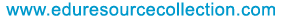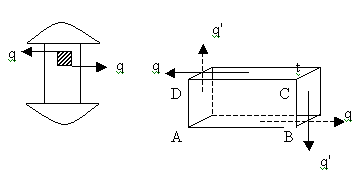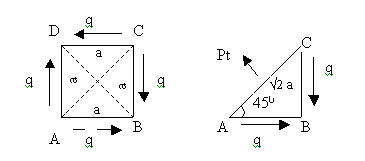@import url(http://www.google.com/cse/api/branding.css);Custom Search The content of the site is exclusively owned byHome | School Level | School Projects | Examinations | Civil | Food Processing | Seminars | Software Testing | Warehousing & Inventory | Networking

# Strength of Materials - Poisson’s Ratio

5. Poisson’s Ratio
Any direct stress is accompanied by a strain in its own direction and called linear strain and an opposite kind strain in every direction at right angles to it, lateral strain. This lateral strain bears a constant ratio with the linear strain. This ratio is called the Poisson’s ratio and is denoted by (1/m) or µ.

Poisson’s Ratio = Lateral Strain / Linear Strain.

Value of the Poisson’s ratio for most materials lies between 0.25 and 0.33.

6. Complementary Strain

Consider a rectangular element ABCD of a body subjected to simple shear of intensity q as shown. Let t be the thickness of the element. Total force on face AB is , FAB = stress X area = q X AB X t. Total force on face CD is, FCD = q X CD Xt = q XAB Xt.FAB and FCD being equal and opposite, constitute a couple whose moment is given by,
M =FAB X BC = q XAB X BC X t

Since the element is in equilibrium within the body, there must be a balancing couple which can be formed only by another shear stress of some intensity q’ on the faces BC and DA. This shear stress is called the complementary stress.
FBC = q’ X BC X t
FDA = q’ X DA X t = q’ X BC Xt
The couple formed by these two forces is M’ = FBC X AB = q’ X BC X t
For equilibrium, M’ = M.
Therefore q’ = q
This enables us to make the following statement.

In a state of simple shear a shear stress of any intensity along a plane is always accompanied by a complementary shear stress of same intensity along a plane at right angles to the plane.

7. Direct Stresses Developed Due to Simple Shear.
Consider a square element of side a and thickness t in a state of simple shear as shown in figure. It is clear that the shear stress on the forces of element causes it to elongate in the direction of the diagonal BD. Therefore a tensile stress of same intensity pt is induced in the elements along BD. ie, across the plane of the diagonal AC. The triangular portion ABC of the element is in equilibrium under the action of the following.(1)FAC = Normal force on face AC = pt X AC X t = pt X v2 aXt
(2)FAB = Tangential force on face AB = q X BC X t = q aXt
(3)FBC = Tangential force on face BC = q X BC X t = q aXt

For equilibrium in the direction normal to AC,
FAC – FAB cos45 – FBC cos45 = 0
Pt X v2 at – q at X 1/v2 - q at X 1/v2 = 0
v2 pt – 2 q /v2 = 0
pt = q

It can also be seen that the shear stress on the faces of the element causes it to foreshorten in the direction of the diagonal BD. Therefore a compressive stress pc is induced in the element in the direction AC, ie across the plane of the diagonal BD. It can also be shown that pc = q.

It can thus be concluded that simple shear of any intensity gives rise to direct stresses of same intensity along the two planes inclined at 45º to the shearing plane. The stress along one of these planes being tensile and that along the other being compressive.